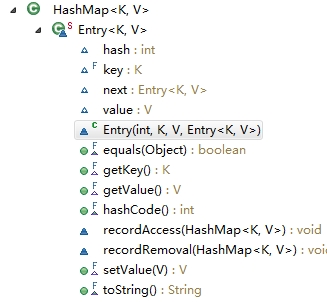# HashMap 和HashSet 源代码详解

HashSet 源代码详解

    public HashSet() {
map = new HashMap<>();
}

    public boolean add(E e) {
return map.put(e, PRESENT)==null;
}

    private static final Object PRESENT = new Object();

    public Iterator<E> iterator() {
return map.keySet().iterator();
}

HashMap 源代码分析：

HashMap概述：
HashMap基于哈希表的 Map 接口的实现。此实现提供所有可选的映射操作，并允许使用 null 值和 null 键。（除了不同步和允许使用 null 之外，HashMap 类与 Hashtable 大致相同。）此类不保证映射的顺序，特别是它不保证该顺序恒久不变。

值得注意的是HashMap不是线程安全的，如果想要线程安全的HashMap，可以通过Collections类的静态方法synchronizedMap获得线程安全的HashMap。

　　Map map = Collections.synchronizedMap(new HashMap());

HashMap的底层实现原理

HashMap的底层主要是基于数组和链表来实现的，它之所以有相当快的查询速度主要是因为它是通过计算散列码来决定存储的位置。HashMap中主要是通过key的hashCode来计算hash值的，只要hashCode相同，计算出来的hash值就一样。如果存储的对象对多了，就有可能不同的对象所算出来的hash值是相同的，这就出现了所谓的hash冲突。学过数据结构的同学都知道，解决hash冲突的方法有很多，HashMap底层是通过链表来解决hash冲突的。HashMap 底层数组中所维护的对象类型： Entry

HashMap 在其内部专门定义了一个类，内部类 Entry类，实现了Map.Entry接口， 其内部还拥有着一些方法，主要包含四个元素，static class Entry<K,V> implements Map.Entry<K,V> {
final K key;
V value;
Entry<K,V> next;//对下一个节点的引用（看到链表的内容，结合定义的Entry数组，是不是想到了哈希表的拉链法实现？！）
final int hash;//哈希值

Entry(int h, K k, V v, Entry<K,V> n) {
value = v;
next = n;
key = k;
hash = h;
}
}

    //几个重要的参数，会大量的用到
static final int DEFAULT_INITIAL_CAPACITY = 1 << 4; // aka 16 默认初始化的大小 为16
static final float DEFAULT_LOAD_FACTOR = 0.75f; // 默认负载因子 为 0.75
int threshold; //扩容的临界值

//HashMap 提供了四种构造方法
//1. 不带参数的构造方法，使用默认值 16 ，0.75
public HashMap() {
}
//2. 带一个参数的构造方法，自己定义Map长度，0.75
public HashMap(int initialCapacity) {
}
//3. 带两个参数的构造方法，为什么会提供这个方法，在之后会讲到，这跟Map的空间和时间效率有关系
public HashMap(int initialCapacity, float loadFactor) {
if (initialCapacity < 0)
throw new IllegalArgumentException("Illegal initial capacity: " +  initialCapacity);
// 在Map中也定义了容量的最大值，跟ArrayList一样
if (initialCapacity > MAXIMUM_CAPACITY)
initialCapacity = MAXIMUM_CAPACITY;
throw new IllegalArgumentException("Illegal load factor: " + loadFactor);

// threshold 为临界值，当集合中元素个数达到时就进行2的指数倍扩容
threshold = initialCapacity;
init();
}
// 将整个集合添加到Map中， 这个过程我们好好分析一下，首先传进来有个Map， 我们可以看到它之后调用了上面的带两个参数的构造函数HashMap(int,float)，第一个数据是 （程序定义的最大容量）和（map的容量/负载因子 +1）中的最大值，比如m.size()=21， 那么初始的容量就为 21/0.75+1=29， 执行HashMap(29,0.75), 临界值 threshold =29；
public HashMap(Map<? extends K, ? extends V> m) {
this(Math.max((int) (m.size() / DEFAULT_LOAD_FACTOR) + 1, DEFAULT_INITIAL_CAPACITY), DEFAULT_LOAD_FACTOR);
// 然后执行是否要扩容的操作，
inflateTable(threshold);
// 将m创建到新的Map中来
putAllForCreate(m);
}
// 扩容函数，为什么数组的长度必须定义为2的整数次幂呢，原因在后面
private void inflateTable(int toSize) {
// Find a power of 2 >= toSize 首先找到一个数据是 2的幂次方 而且 大于toSize， 传进来的是29 意味着得到的capacity 是32
int capacity = roundUpToPowerOf2(toSize);
// 修改扩容的临界值，根据传进来的值，临界值为24，
threshold = (int) Math.min(capacity * loadFactor, MAXIMUM_CAPACITY + 1);
// 申请一个32 个空间大小的Entry数组,table就是一个Entry类型的数组
table = new Entry[capacity];
// 初始化需要的hash种子
initHashSeedAsNeeded(capacity);
}


    public V put(K key, V value) {
//首先判断是否为一个空表，若为空表则分配空间
if (table == EMPTY_TABLE) {
inflateTable(threshold);
}
// 如果key是Null的时候执行自己的算法，因此可见，HashMap是允许存放Null键和Null值的
if (key == null)
return putForNullKey(value);
// Key不为空的情况
// 首先根据key值计算出一个hash值
int hash = hash(key);
// 根据hash值计算出在table中的下标
int i = indexFor(hash, table.length);
// 判断是否发生了地址冲突
for (Entry<K,V> e = table[i]; e != null; e = e.next) {
Object k;
// 如果是相同的Key值，则进行覆盖，返回旧的value值
if (e.hash == hash && ((k = e.key) == key || key.equals(k))) {
V oldValue = e.value;
e.value = value;
e.recordAccess(this);
return oldValue;
}
}
modCount++;
// 添加一个新的Entry对象到数组中去
addEntry(hash, key, value, i);
}

Key值为空的时候执行了 putForNullKey(value)，我们看了源代码可以发现，它直接将其放在数组中下标为0的位置，并没有计算hash值，这根地址计算方式有关系，因为所有的Null键都是放在数组中下标为table的位置的，我们需要记住。

    private V putForNullKey(V value) {
for (Entry<K,V> e = table; e != null; e = e.next) {
if (e.key == null) {
V oldValue = e.value;
e.value = value;
e.recordAccess(this);
return oldValue;
}
}
modCount++;
addEntry(0, null, value, 0);
return null;
}

    final int hash(Object k) {
int h = hashSeed;
if (0 != h && k instanceof String) {
return sun.misc.Hashing.stringHash32((String) k);
}

h ^= k.hashCode();

h ^= (h >>> 20) ^ (h >>> 12);
return h ^ (h >>> 7) ^ (h >>> 4);
}

    static int indexFor(int h, int length) { //根据hash值和数组长度算出索引值
return h & (length-1);  //这里不能随便算取，用hash&(length-1)是有原因的，这样可以确保算出来的索引是在数组大小范围内，不会超出
}

假设数组长度分别为15和16，优化后的hash码分别为8和9，那么&运算后的结果如下：
h & (table.length-1) hash table.length-1
8 & (15-1)： 0100 & 1110 = 0100
9 & (15-1)： 0101 & 1110 = 0100
———————————————————————————————————————–
8 & (16-1)： 0100 & 1111 = 0100
9 & (16-1)： 0101 & 1111 = 0101

    void addEntry(int hash, K key, V value, int bucketIndex) {
// 是否要扩容
if ((size >= threshold) && (null != table[bucketIndex])) {
resize(2 * table.length);
hash = (null != key) ? hash(key) : 0;
bucketIndex = indexFor(hash, table.length);
}

createEntry(hash, key, value, bucketIndex);
}
// 创建一个新的Entry对象，我们可以看到原来该位置上的旧的对象作为新的对象的Next属性被添加到Entry数组中，table[bucketIndex]指向了新的对象，也就意味这新的对象被加到了该位置上的链表的首部。
void createEntry(int hash, K key, V value, int bucketIndex) {
Entry<K,V> e = table[bucketIndex];
table[bucketIndex] = new Entry<>(hash, key, value, e);
size++;
}

    void resize(int newCapacity) {
Entry[] oldTable = table;
int oldCapacity = oldTable.length;
// 这个if块表明，如果容量已经到达允许的最大值，即MAXIMUN_CAPACITY，则不再拓展容量，而将装载拓展的界限值设为计算机允许的最大值。
// 不会再触发resize方法，而是不断的向map中添加内容，即table数组中的链表可以不断变长，但数组长度不再改变
if (oldCapacity == MAXIMUM_CAPACITY) {
threshold = Integer.MAX_VALUE;
return;
}
// 创建新数组，容量为指定的容量
Entry[] newTable = new Entry[newCapacity];
transfer(newTable, initHashSeedAsNeeded(newCapacity));
table = newTable;
threshold = (int)Math.min(newCapacity * loadFactor, MAXIMUM_CAPACITY + 1);
}

// 将原数组中的对象重新计算数组下标，并复制到新的数组中去
void transfer(Entry[] newTable, boolean rehash) {
int newCapacity = newTable.length;
for (Entry<K,V> e : table) {
while(null != e) {
Entry<K,V> next = e.next;
if (rehash) {
e.hash = null == e.key ? 0 : hash(e.key);
}
int i = indexFor(e.hash, newCapacity);
// 将e插入到newTable[i]指向的链表的头部
e.next = newTable[i];
newTable[i] = e;
e = next;
}
}
}

  public V get(Object key) {
if (key == null)
return getForNullKey();
Entry<K,V> entry = getEntry(key);

return null == entry ? null : entry.getValue();
}
// 获取根据下标获取Entry对象
final Entry<K,V> getEntry(Object key) {
if (size == 0) {
return null;
}

int hash = (key == null) ? 0 : hash(key);
for (Entry<K,V> e = table[indexFor(hash, table.length)];
e != null;
e = e.next) {
Object k;
if (e.hash == hash &&
((k = e.key) == key || (key != null && key.equals(k))))
return e;
}
return null;
}

    public void clear() {
modCount++;
Arrays.fill(table, null); // 调用了数组的处理方法，全部置为空
size = 0;
}

    private transient Set<Map.Entry<K,V>> entrySet = null;

public Set<Map.Entry<K,V>> entrySet() {
return entrySet0();
}

private Set<Map.Entry<K,V>> entrySet0() {
Set<Map.Entry<K,V>> es = entrySet;
return es != null ? es : (entrySet = new EntrySet());
}

values()方法，keySet()方法也是同样的实现原理。

    private final class EntrySet extends AbstractSet<Map.Entry<K,V>> {
public Iterator<Map.Entry<K,V>> iterator() {
return newEntryIterator();
}
public boolean contains(Object o) {
if (!(o instanceof Map.Entry))
return false;
Map.Entry<K,V> e = (Map.Entry<K,V>) o;
Entry<K,V> candidate = getEntry(e.getKey());
return candidate != null && candidate.equals(e);
}
public boolean remove(Object o) {
return removeMapping(o) != null;
}
public int size() {
return size;
}
public void clear() {
HashMap.this.clear();
}
}